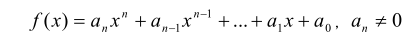# P1067 多项式输出

• 20.8K通过
• 73K提交
• 题目提供者 CCF_NOI
• 评测方式 云端评测
• 标签 字符串 数论,数学 NOIp普及组 2009
• 难度 普及-
• 时空限制 1000ms / 128MB
• 提示：收藏到任务计划后，可在首页查看。

## 题目描述

一元$n$次多项式可用如下的表达式表示：其中，$a_ix^i$称为$i$次项，$a_i$ 称为$i$次项的系数。给出一个一元多项式各项的次数和系数，请按照如下规定的格式要求输出该多项式：

1. 多项式中自变量为$x$，从左到右按照次数递减顺序给出多项式。

2. 多项式中只包含系数不为$0$的项。

3. 如果多项式$n$次项系数为正，则多项式开头不出现“$+$”号，如果多项式$n$次项系

数为负，则多项式以“$-$”号开头。

4. 对于不是最高次的项，以“$+$”号或者“$-$”号连接此项与前一项，分别表示此项

系数为正或者系数为负。紧跟一个正整数，表示此项系数的绝对值（如果一个高于$0$次的项，

其系数的绝对值为$1$，则无需输出 $1$）。如果$x$的指数大于$1$，则接下来紧跟的指数部分的形

式为“$x^b$”，其中 $b$为 $x$的指数；如果 $x$的指数为$1$，则接下来紧跟的指数部分形式为“$x$”；

如果 $x$ 的指数为$0$，则仅需输出系数即可。

5. 多项式中，多项式的开头、结尾不含多余的空格。

## 输入输出格式

输入格式：

输入共有 $2$ 行

第一行$1$ 个整数，$n$，表示一元多项式的次数。

第二行有 $n+1$个整数，其中第$i$个整数表示第$n-i+1$ 次项的系数，每两个整数之间用空格隔开。

输出格式：

输出共 $1$ 行，按题目所述格式输出多项式。

## 输入输出样例

输入样例#1： 复制
5
100 -1 1 -3 0 10
输出样例#1： 复制
100x^5-x^4+x^3-3x^2+10
输入样例#2： 复制
3
-50 0 0 1

输出样例#2： 复制
-50x^3+1


## 说明

NOIP 2009 普及组 第一题

对于100%数据，$0 \le n \le 100$,$-100 \le$系数$\le 100$

提示
标程仅供做题后或实在无思路时参考。
请自觉、自律地使用该功能并请对自己的学习负责。
如果发现恶意抄袭标程，将按照I类违反进行处理。